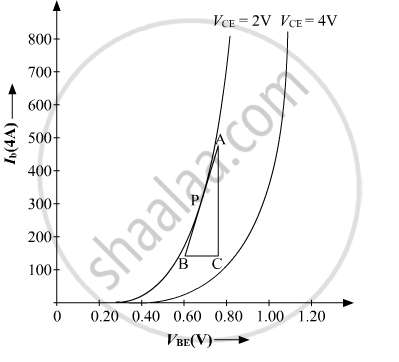# Input Resistance How Are These Determined Using Typical Input and Output Characteristics? - Physics

Define the terms : Input resistance How are these determined using typical input and output characteristics?

#### Solution

Input resistance may be defined as the ratio of small change in the base-emitter voltage (ΔVBE) to the resulting change in base current (IB) at constant collector-emitter voltage (VCE).

Input resistanceR_i=(DeltaV_(BE))/(DeltaI_B)To find the input resistance, mark a point P on the input characteristic. Now, draw a tangent at point P. The reciprocal of slope of AB will give the input resistance

Concept: Junction Transistor - Transistor as an Amplifier (Ce-configuration)
Is there an error in this question or solution?
2014-2015 (March) Delhi Set 1

Share